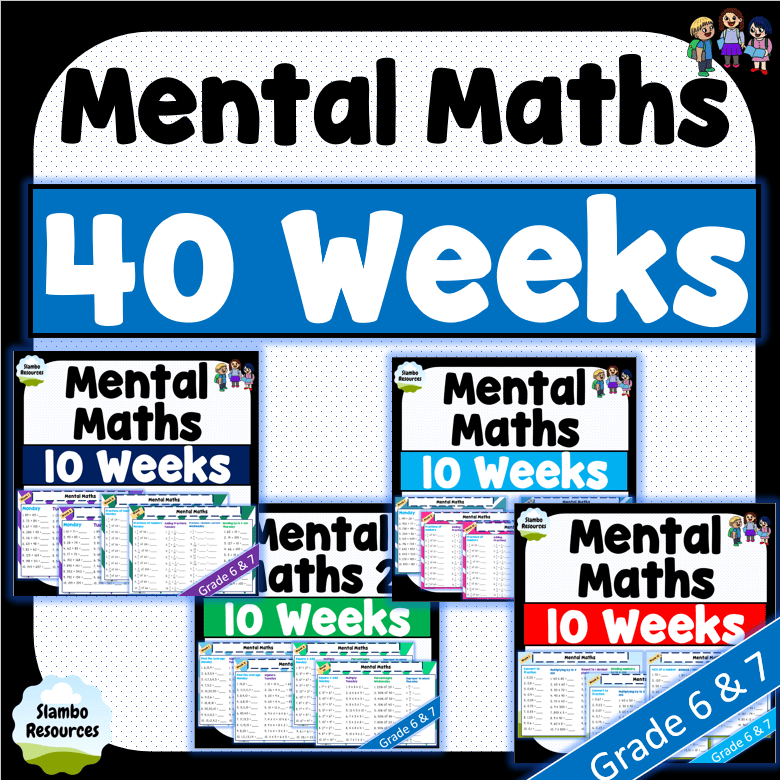# Grade 6 Mental Math Worksheets

Free grade 6 mental math worksheets to help your students develop  their mental math skills! These free worksheets are designed to give students repetition in addition, subtraction, multiplication and division. The ability to calculate problems quickly and accurately in Mathematics is important. The resources below also have the students working on multiplying and dividing by 10 and 100, which is important to understand in grade 6. Challenge your students to time themselves each day and see if they can improve. These worksheets are great for daily math warm ups or homework! I hope you find them helpful. For loads and loads of mental math worksheets, have a look around my TpT store by clicking on the images below.

### Mental Math Worksheets### Bundle

A bundle of the 4 resources.

## Multiplying by 10, 100 and 1000

The worksheets above test the students’ ability to multiply by 10 and 100. This is quite simple, it just takes practice. These questions should be answered mentally and are easier for students who have fast time table recall.

Strategy: The strategy I teach my class is to ignore the zeros and multiply the numbers, and then add the zeros back to your answer. Simple!

Example 1: 40 x 80 – Ignore the 2 zeros. 4 x 8 = 32.